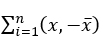# ICSE Worksheet for chapter-15 Statistics class 9

## Find ICSE Worksheet for chapter-15 Statistics class 9

CLASS-9

BOARD: ICSE

Mathematic Worksheet - 15

TOPIC: Statistics

For other ICSE Worksheet for class 9 Mathematic check out main page of Physics Wallah.

#### OBJECTIVE

1. If the arithmetic mean of 5,7, 9,x is 9 then the value of x is
1. 11
2. 15
3. 18
4. 16
2. If the mean and median of a set of nos. are 8 and 9 respectively then the mode will be
1. 7.2
2. 8.2
3. 9.2
4. 10.2
3. A student got marks in 5 subjects in a monthly test is given below:
2, 3,4,5,6, in these obtained marks 4 is the
1. Mean and Median
2. Median but no mean
3. Mean but not the median
4. Mode
4. What is the mode from the following table?
 Marks obtained 3 1 23 33 43 frequency 7 11 15 8 3
1. a) 13
2. b) 43
3. c) 33
4. d) 23
5. Which one of the following is not correct-?
1. Statistics is liable to be misused
2. The data collected by the investigator to be used by himself are called primary data
3. Statistical laws are exact
4. Statistics do not take into account of individual cases.
6. The following no. are given 61 62 63 61 63 64 64 60 65 63 64 65 66 64 the difference between their mean and median is
1. 0.4
2. 0.3
3. 0.2
4. 0.1
7. The value ofwhere is the arithmetic mean of xi is
1. a) 1
2. b) n
3. c) 0
4. d) None of these
8. The average of 15 numbers is 18.The average of first 8 is 9 and that last is 17 then the 8th number is
1. 15
2. 16
3. 18
4. 20

#### SUBJECTIVE

1. 1) Find the median of
2. i) 5 30 15 6 18 22 26 32 6 9 18
3. ii) 92 88 62 53 55 59 60 61 85 89
4. iii) 66 69 108 72 78 82 98 99 102 101
5. 2).  Find the missing frequencies of the following distribution if its known that the mean of the distribution is 50.
6.
7. X : 10 30 50 70 90 total
8. F : 17 f1 32 f2 19 120
9. 3.  Find the mean for the following data
 Age (years) 25-30 30-35 35-40 40-45 45-50 50-55 No. of teachers 30 23 20 14 10 3
10. The mean of a certain group of observation is 78. Find the resulting mean if the value of each observation is:
1. increased by 2
2. decreased by 3
3. multiplied by 1.5
4. divided by 2
5. increased by 30 %
6. diminished by 25%
11. Draw a histogram to represent the following data:
 Class interval 40-60 60-80 80-100 100-120 120-140 140-160 160-180 180-200 Frequency 20 40 30 50 30 20 10 40
12. Draw a bar graph to represent the following:

 A B C D E F 60 70 55 40 90 50

Solutions: to worksheet-15 Topic-Statistics

1. b
2. c
3. a
4. d
5. c
6. b
7. c
8. c

1. 18,
2. 61.5,
3. 90
1. 28, 24
2. 35.5
1. 80,
2. 75,
3. 117,
4. 39,
5. 101.4,
6. 58.5